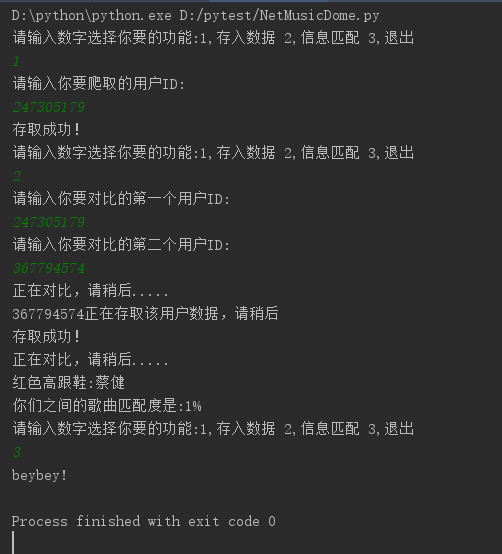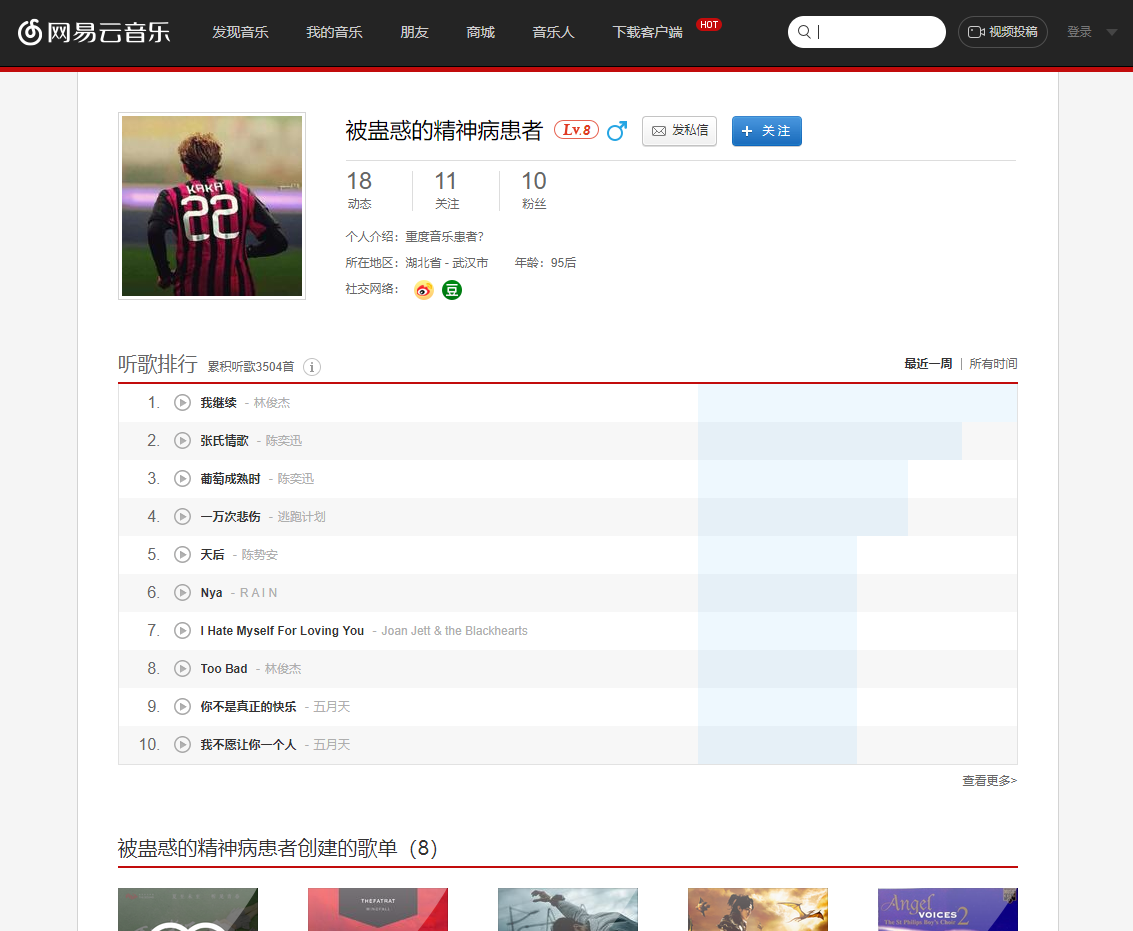## 网易云音乐听歌排行

### 功能

• 爬取用户所有时间的听歌排行
• 用户之间的数据匹配

### 分析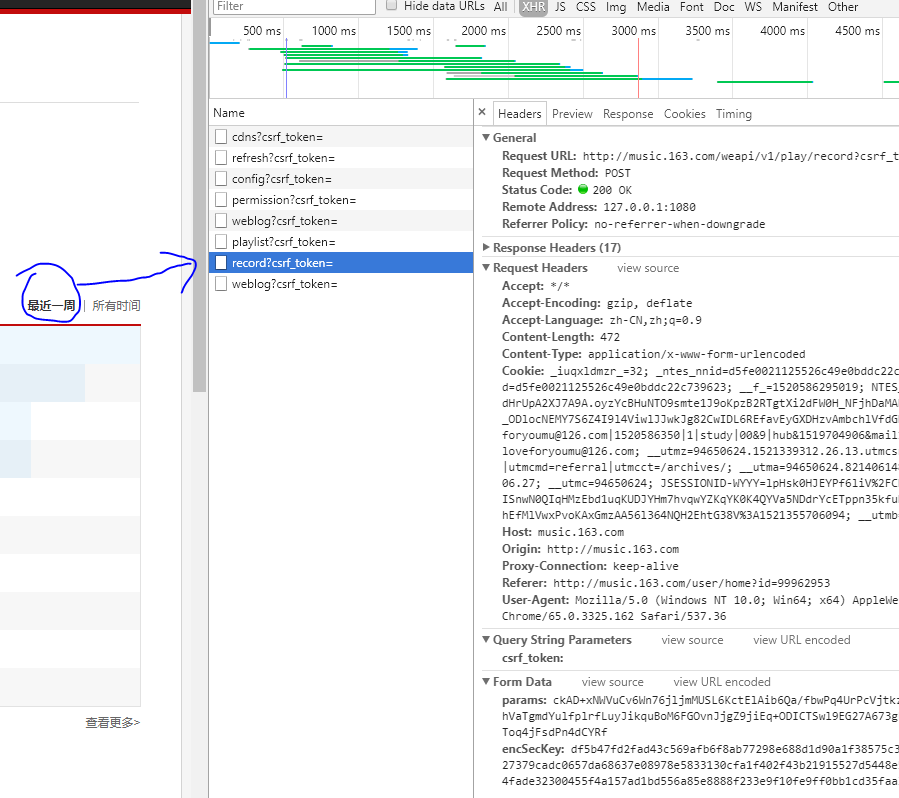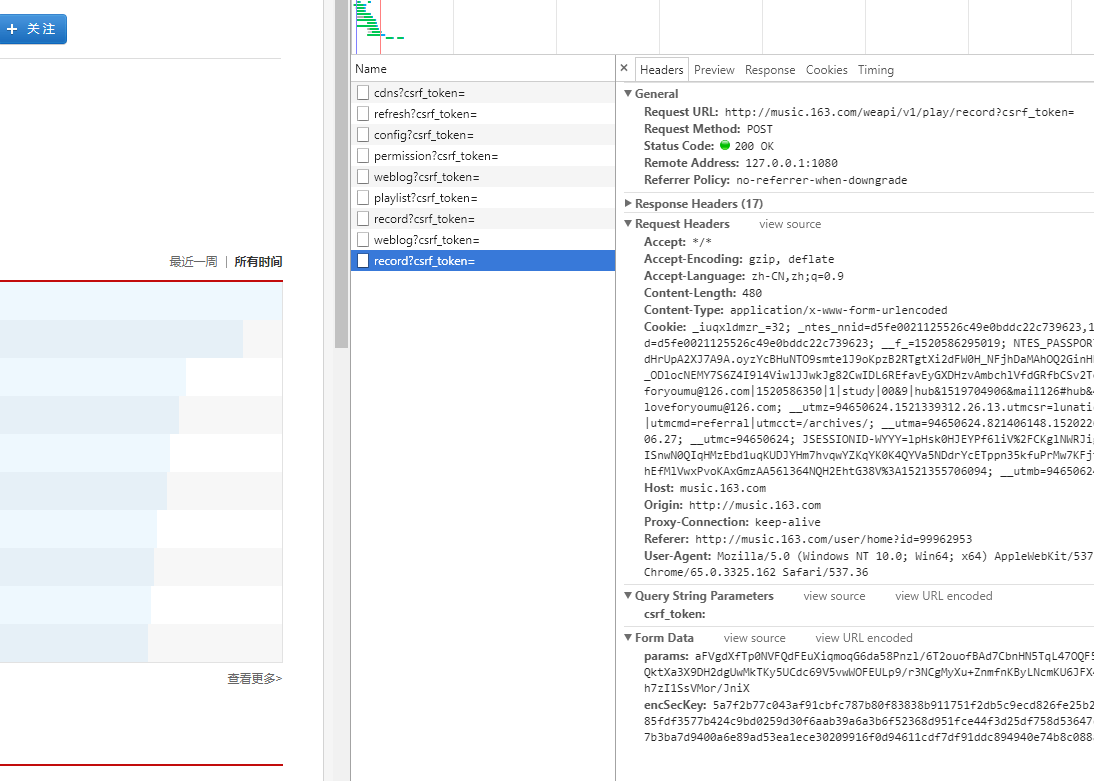### 功能实现

#### 爬取听歌排行

##### 爬取听歌排行代码
``````def Work_Mian(id, state=1 , id1=1):
chrome_options = webdriver.ChromeOptions()
chrome_options.binary_location ="H:\Program Files (x86)
\Chrome\Application\chrome.exe"
# 声明浏览器
a = webdriver.Chrome(chrome_options=chrome_options)
# 爬取地址
url = 'http://music.163.com/#/user/songs/rank?id='
url = url+id
try:
a.get(url)
# 从window转到ifram
a.switch_to.frame('g_iframe')
# 显式等待声明
wait = ui.WebDriverWait(a, 15)
# 等待 a.find_element_by_class_name('g-bd') 记载
if wait.until(lambda a: a.find_element_by_class_name('g-bd')):
# 判断是否有"所有时间"按钮
if a.find_element_by_xpath('//*[@id="songsall"]'):
# 点击所有时间按钮，页面的ajax同步所有时间的结果
a.find_element_by_xpath('//*[@id="songsall"]').click()
# 强制等待 让界面刷新等待数据出现
time.sleep(0.2)
# 同上
if wait.until(lambda a: a.find_element_by_class_name('g-bd')):
# print(type(a.page_source))
# print(a.page_source)
# 声明正则表达式
pattern = re.compile('<b title=".*?">(.*?)</b>.*?hidefo
cus="true">(.*?)</a>.*?style="width:(.*?);"', re.S)
gorp = re.findall(pattern, a.page_source)
# 将读取到的数据存入文本文档
for i in gorp:
f = open("song/"+id+'.txt', 'a+', encoding='utf-8')
f.write(i+':'+i.replace('\xa0', ' ')+'   '+i+'\n')
f.close()
print('存取成功！')
a.quit()
if state == 1:
main()
else:
Net_Compare.compare(id, id1)

except NoSuchElementException:
if state == 1:
print('ID输入错误，请重新输入！')
a.close()
input_id()
if state == 2:
print(str(id)+'该用户数据被设为隐私，不可爬取')
main()
``````

``````a = webdriver.Chrome('xxxx')
# xxx改成你下载chrome驱动的位置，一般把下载好的放到chrome的安装目录中。
``````

#### 数据匹配对比

###### 匹配对比代码
``````def ztt(id , id1):
try:
ztt = codecs.open('song/'+id+'.txt', 'r', encoding='UTF-8')

except IOError:
print(str(id)+'正在存取该用户数据，请稍后')
NetMusicDome.Work_Mian(id, 2, id1)
ztt = codecs.open('song/' + id + '.txt', 'r', encoding='UTF-8')
else:

for i in range(len(content)):
content[i] = content[i][:-8]
return content

def compare(id1, id2):
print('正在对比，请稍后.....')
one = ztt(id1, id2)
two = ztt(id2, id1)
sum = 0
try:
for z in one:
for y in two:
if z == y:
sum = sum + 1
print(z)
except TypeError:
a = 0
else:
print('你们之间的歌曲匹配度是:' + str(sum) + '%')
``````

### 整体代码

#### NetMusicDome.py

``````from selenium import webdriver
import selenium.webdriver.support.ui as ui
import time
import re
import Net_Compare
from selenium.common.exceptions import NoSuchElementException
"""

1,先用显式浏览器在测试代码
3,放置服务器进行设置
"""

def Work_Mian(id, state=1 , id1=1):
chrome_options = webdriver.ChromeOptions()
chrome_options.binary_location ="H:\Program Files (x86)\
Chrome\Application\chrome.exe"
# 声明浏览器
a = webdriver.Chrome(chrome_options=chrome_options)
# 爬取地址
url = 'http://music.163.com/#/user/songs/rank?id='
url = url+id

try:
a.get(url)
# 从window转到ifram
a.switch_to.frame('g_iframe')
# 显式等待声明
wait = ui.WebDriverWait(a, 15)
# 等待 a.find_element_by_class_name('g-bd') 记载
if wait.until(lambda a: a.find_element_by_class_name('g-bd')):
# 判断是否有"所有时间"按钮
if a.find_element_by_xpath('//*[@id="songsall"]'):
# 点击所有时间按钮，页面的ajax同步所有时间的结果
a.find_element_by_xpath('//*[@id="songsall"]').click()
# 强制等待 让界面刷新等待数据出现
time.sleep(0.2)
# 同上
if wait.until(lambda a: a.find_element_by_class_name('g-bd')):
# print(type(a.page_source))
# print(a.page_source)
# 声明正则表达式
pattern = re.compile('<b title=".*?">(.*?)</b>.*?
hidefocus="true">(.*?)</a>.*?style="width:
(.*?);"', re.S)
gorp = re.findall(pattern, a.page_source)
# 将读取到的数据存入文本文档
for i in gorp:
f = open("song/"+id+'.txt', 'a+', encoding='utf-8')
f.write(i+':'+i.replace('\xa0', ' ')+'   '+i+'\n')
f.close()
print('存取成功！')
a.quit()
if state == 1:

main()
else:
Net_Compare.compare(id, id1)

except NoSuchElementException:
if state == 1:
print('ID输入错误，请重新输入！')
a.close()
input_id()
if state == 2:
print(str(id)+'该用户数据被设为隐私，不可爬取')
main()

def input_id():
print('请输入你要爬取的用户ID:')
id = input()
Work_Mian(id)
main()

def Compare():
print('请输入你要对比的第一个用户ID:')
one = input()
print('请输入你要对比的第二个用户ID:')
two = input()
Net_Compare.compare(one, two)
main()

def main():
print('请输入数字选择你要的功能:1,存入数据 2,信息匹配 3,退出')
a = input()
if a == str(1):
input_id()
if a == str(2):
Compare()
if a == str(3):
print('beybey!')
exit()
if a != str(2) and a !=str(1) and a!=str(3):
print('输入错误，请重新输入！')
main()
if __name__ == '__main__':
main()
``````

#### Net_Compare.py

``````# 无聊写一个对比歌曲重合度的一个小Dome
import codecs
import NetMusicDome

def ztt(id , id1):
try:
ztt = codecs.open('song/'+id+'.txt', 'r', encoding='UTF-8')

except IOError:
print(str(id)+'正在存取该用户数据，请稍后')
NetMusicDome.Work_Mian(id, 2, id1)
ztt = codecs.open('song/' + id + '.txt', 'r', encoding='UTF-8')
else:

for i in range(len(content)):
content[i] = content[i][:-8]
return content

def compare(id1, id2):
print('正在对比，请稍后.....')
one = ztt(id1, id2)
two = ztt(id2, id1)
sum = 0
try:
for z in one:
for y in two:
if z == y:
sum = sum + 1
print(z)
except TypeError:
a = 0
else:
print('你们之间的歌曲匹配度是:' + str(sum) + '%')
``````

### Output

#### 音乐信息存取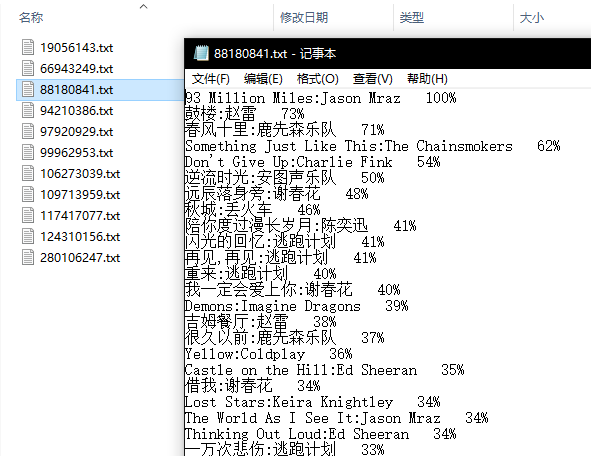#### 匹配对比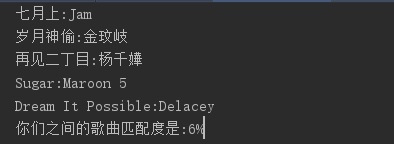#### Dome展示